# Linnart

Data scientist focusing on simulation, optimization and modeling in R, SQL, VBA and Python

## Lambda functions in PythonA quick introduction to lambda functions in Python. Below you find some examples of lambda functions in Python. Documentation is added to the code directly – in the form of comments. Lambda fucntions in Python are stated in accordance with […]

## Basic animations with Matplotlib in PythonI want to provide a brief example on how you can create plot-based animations with Matplotlib in Python. Below Python code implements a simple exponential growth animation. Documentation is added directly to the code in the form of comments. # […]

## Developing a simple agent-based simulation model in PythonIn a previous post I demonstrated how to visualize a 2D grid, using matplotlib and pyplot in Python (post titled “Visualizing 2D grids and arrays using matplotlib in Python”). That post was meant as first introduction on how to visualize […]

## Visualizing 2D grids and arrays using matplotlib in Python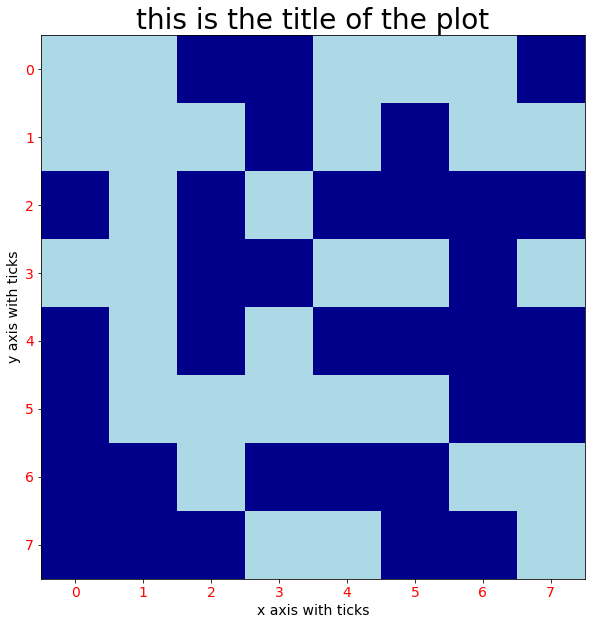A 2D grid array plot can be a valuable visualization tool, e.g. in the area of agent-based simulation. In this post I want to give a brief tutorial in how you can visualize a 2D grid array, using matplotlib in […]

## List comprehensions in PythonThis post is a part of my Python documentation, in case you are learning Python. In below coding examples I cover list comprehensions, a compact way of creating lists, allowing for filtering too. # first example of a python list […]

## Introduction to NumPy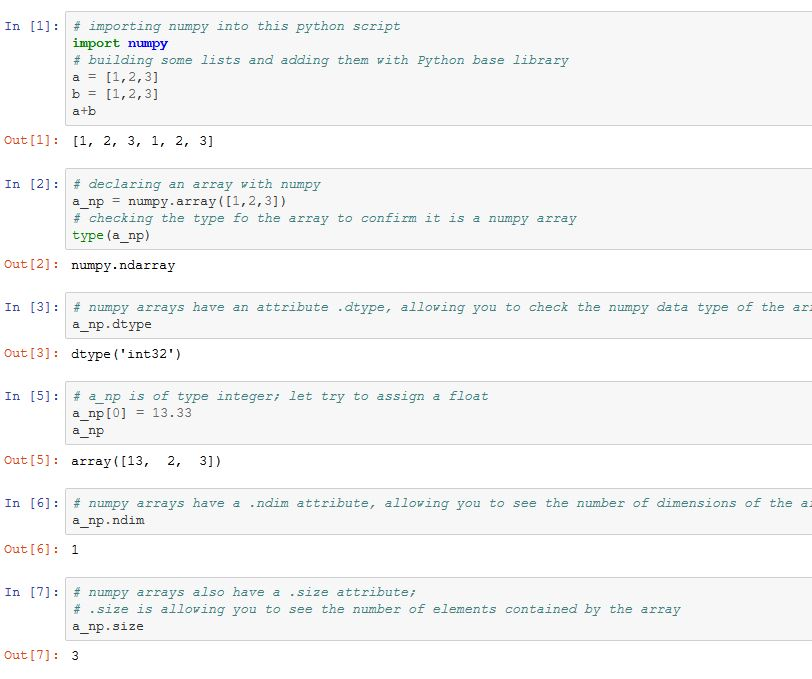This post provides an introductionary documentation for getting started with NumPy in Python. # importing numpy into this python script import numpy # building some lists and adding them with Python base library a = [1,2,3] b = [1,2,3] a+b […]

## Introduction to Matplotlib in PythonBesides Pandas and Seaborn I would also like to provide you with a brief introduction to matplotlib. Matplotlib is another important module and library in Python. It is used for data visualization. Below coding example will get you started. The […]

## Introduction to Seaborn in Python, used with Pandas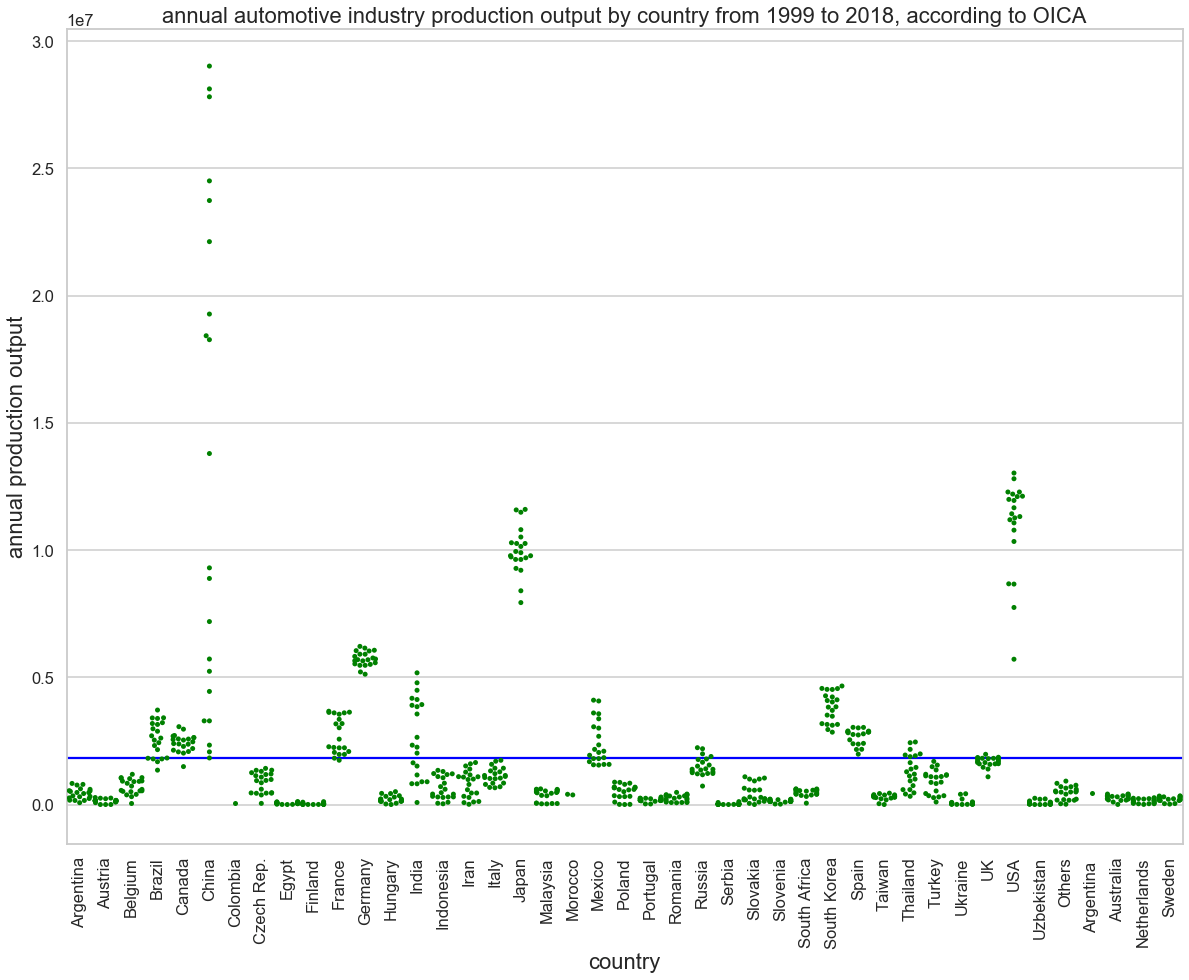This post is a rough introduction to the Seaborn module in Python. I use it for data visualization, in combination with Pandas. Read the comments to understand my workflow. Any questions you can leave as a comment at the bottom […]

## Introduction to Pandas in PythonThis is an introduction to the Pandas module in Python. I demonstrate core Pandas functionality using a tabular dataset from OICA. # import pandas import pandas # read in oica.csv file with data pandas.read_csv(“oica.csv”,sep=”,”) year country output 0 2018 Argentina […]

## Implementing a random walk forecast in Python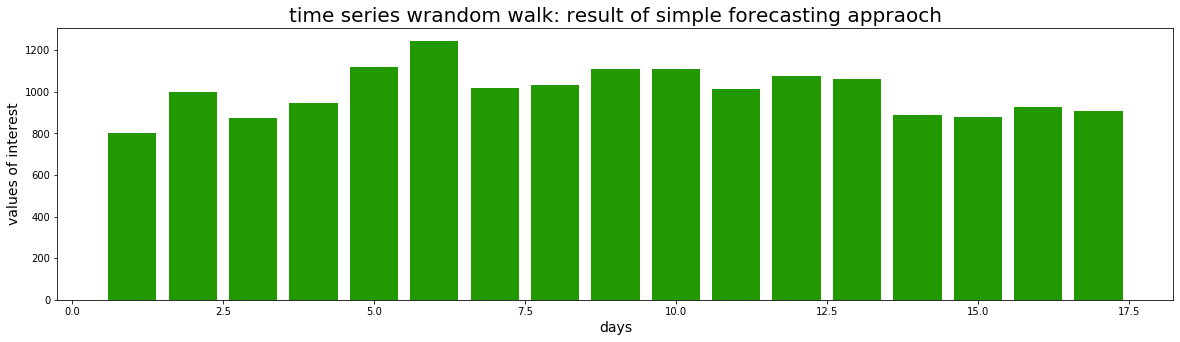In previous posts I introduced very simple (and naive) forecasting methods, namely CAGR-based forecasting and simple moving average forecasting. I implemented such forecasting methods in R and demonstrated basic use cases. In this post I want to introduce another simple […]

## Forecasting US automotive industry production output with simple moving averageIn a previous post I explained CAGR-based forecasting. CAGR-based forecasting is a very simple forecasting method which is often applied in industry, e.g. for forecasting sales and production output. Simple forecasting models have benefits. They are easy to understand and easy […]

## CAGR-based forecasting, using OICA production data (in R)In this post I provide an example of CAGR-based forecasting, using OICA vehicle production data for Chinese automotive industry. CAGR is the compounded average growth rate. If e.g. production output in year 2000 is 1,000,000 units then, if CAGR = […]

## An (in-complete) overview of some public data sources related to automotive industryOICA production output statistics: OICA is the International Organization of Motor Vehicle Manufacturers. OICA provides sales and production statistics for all relevant countries in open data sets. These can be retrieved from http://www.oica.net/category/production-statistics/ EPA data: EPA is the United States […]

## Testing “coronavirus”, a package in R for accessing data from J.H. university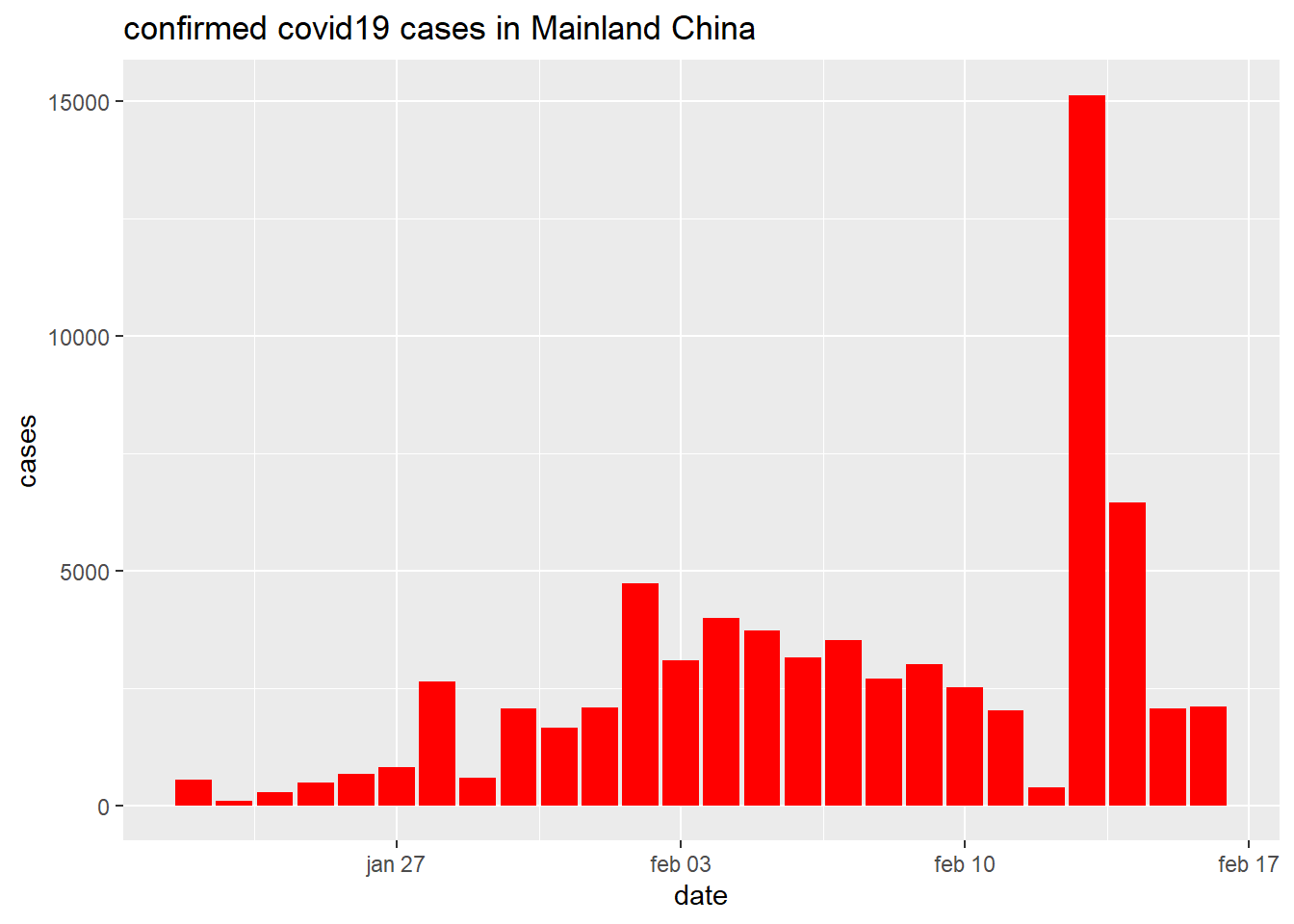This is a just small post where I share some experiment I did with the coronavirus package in R. It provides access to data shared by Johns Hopkins University. Unfortunately, at the moment of this post the latest data entries […]

## OICA production output statistics for automotive industry (R analysis)In this post I want to show another public data source related to automotive industry. OICA, the International Organization of Motor Vehicle Manufacturers, provides a series of statistics on its website, including sales and production statistics. The comprises all manufacturers […]